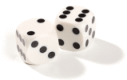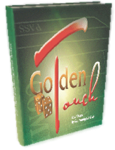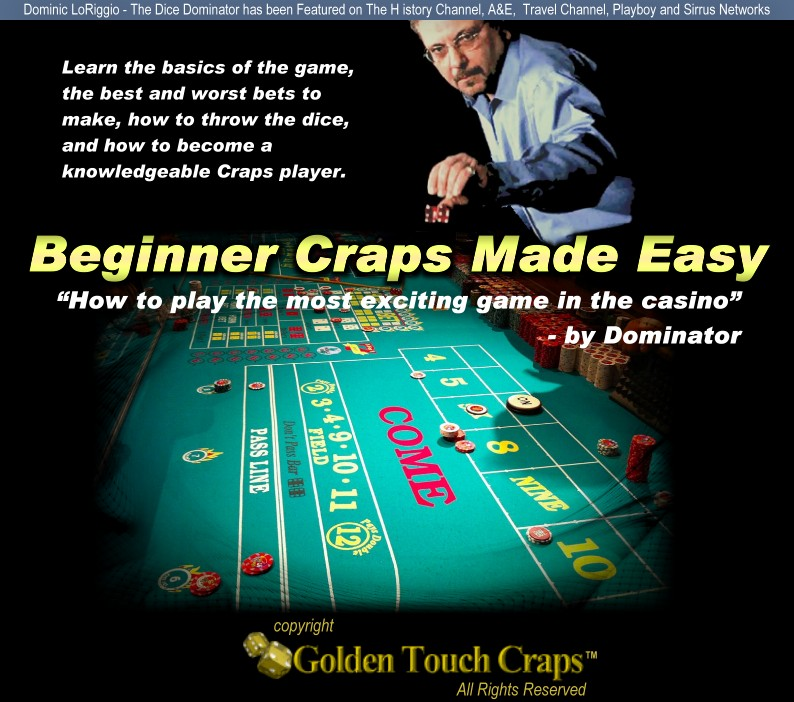# Learn What Dice Sets Are Optimum For Playing At The Craps Table

"As published in Blackjack Insider (www.bjinsider.com) [linked]"

Optimal Dice Set for Dice Controllers in Craps

By Dan Pronovost

Dan Pronovost is the owner and president of DeepNet Technologies, makers of a wide range of gambling training products and software. Their web sites are: www.HandheldBlackjack.com and www.SmartCraps.com and all products are available for free trial download. Dan is the creator of the new card counting system Speed Count, which is being taught by Henry Tamburin and Frank Scoblete in the Golden Touch™ Blackjack two day courses: www.GoldenTouchBlackjack.com.

Introduction

In my craps article last month, I continued introducing readers to Smart Craps, a new software program and statistical analysis tool for dice controllers in the game of craps. We saw in the first article that the seven to rolls ratio (SRR), while somewhat intuitive, is not the best possible measure of dice setting skill. This results from the fact that sevens can be both 'good' and 'bad', meaning that they occur when we both achieve and fail z-axis control. This weakens the statistical utility of SRR for determining player dice setting skill. In the second article, we learned about Pro Test… a new and more powerful statistical analysis tool for analyzing whether you are influencing the dice outcomes.

This month, we'll see that not only can we use Pro Test to determine if we are good dice controllers (instead of just lucky), but also to determine our actual edge in the game, and optimal dice sets. If you haven't yet read the prior two articles, I recommend you do so before continuing below:

Pro Test Player Edge

So… you've practiced your dice control, recorded a few hundred throws, and pass some (or all) of the Pro Tests. Does this mean you're going to make a killing at the casino? What is your player edge (or expectation) on different bets?

While not immediately obvious, it is possible to convert Pro Test results into game expectations. To do so, you need to know:

• The rules of the craps game you are playing in, such as the odds and pay schedule.
• The specific bets you are going to make.
• The dice sets you will use at each point in the throwing cycle.

Smart Craps has two ways for you to determine your edge in the game:

1) Exact mathematical analysis for given simple bets (such as pass, or don't pass, with or without odds).

2) Craps game simulation.

Converting Pro Test results to exact player expectation

Normally for a random shooter, each of the 36 dice outcomes has exactly 1 in 36 chance of occurring. For dice setters, the odds will vary for each potential outcome in a predictable manner. Once we have a combination of Pro Test results (a shooter may only pass one or two tests, or possibly all three), these can be converted into specific probabilities for each of the 36 outcomes. Once we have this and the dice sets used at every point in the game, we can determine the probabilities for each dice sum. And with this knowledge, we can determine the actual player edge given a specific betting pattern and game. We can do this mathematically, without empirical simulation.

Suppose a shooter passes all three Pro Tests with results p1, p2 and p3 in n rolls (this analysis can be applied similarly if a shooter passes any combination of Pro Tests). For the moment, ignore what the actual dice set is, and think of the outcomes as being in one of the following groups:

1) Pro 1 failures: for any dice set, we know there are 4 * 4 = 16 Pro 1 passing outcomes. Therefore, there are 36 - 16 = 20 possible Pro 1 failure outcomes.

2) Pro 1 passes, excluding Pro 2 and 3: We know there are 16 Pro 1 passes, and 4 each of the Pro 2 passes and Pro 3 failures. This leaves 16 - 8 = 8 Pro 1 passes that are not Pro 2 passes and Pro 3 failures. These are the single pitches (Pro 2 and 3 are zero and double pitches respectively).

3) Pro 2 passes: There are exactly 4 Pro 2 passes of the 16 Pro 1 passes (the hardway results: 22, 33, 44, 55).

4) Pro 3 failures: There are exactly 4 Pro 3 failures (the 'good' seven sums: 25, 52, 34, 43).

For each group above, the outcomes in that group each have the same probability of occurring. For a random throw, this would be exactly 1 in 36. But for a controlled shooter, it will vary.

If a shooter passes the Pro 1 test with a value of p1 in n rolls (such as 57 in 100 throws, in the prior example), then we can assume that the probability of all of the outcomes in the first group is 1 - p1/n. Later, we'll look more closely at this assertion, and add a further conservative confidence interval that weakens the Pro test values, even with a passing result. But for simplicity, we can guess that the shooter's actual talent leads to a probability of Pro 1 failure as noted above. For a random shooter, p1/n is 16/36, so 1 - p1/n = 1 - 16/36 = 5/9 = 55.56%.

For a controlled shooter (say, 57 Pro 1 Passes in 100 rolls), the probability of Pro 1 failure is: 1 - (57/100) = 43/100 = 43.00%. This is significantly less than the random shooter (55.56%, above).

If a shooter passes the Pro 2 test with a value of p2 in p1 Pro 1 passes (such as 23 in 57 Pro 1 passes, as we saw earlier), then the probability of the outcomes in the third group is: (p2/p1)*(p1/n) = p2/n. For a random shooter, p2/n is 4/36 = 1/9 = 11.11%.

For a controlled shooter (say, 23 Pro 2 passes in 57 Pro 1 passes), the probability of all Pro 2 passes is 23/100 = 23.00%. This is significantly higher than the random shooter.

If a shooter passes the Pro 3 test with a value of p3 in p1 Pro 3 failures (such as 6 in 57 Pro 1 passes, as we saw earlier), then the probability of the outcomes in the forth group is: (p3/p1)*(p1/n) = p3/n. For a random shooter, p3/n is 4/36 = 1/9 = 11.11%.

For a controlled shooter (say, 6 Pro 3 failures in 57 Pro 1 passes), the probability of all Pro 3 failures is 6/100 = 6.00%. This is significantly lower than the random shooter.

Now, armed with this approach and a given dice set, we can exactly state the probability of each of the 36 outcomes for a controlled shooter. This is not a simple mathematical operation to complete by hand, by is easy to do with a computer and a bit of code (and this is what Smart Craps does). Then, we can simply sum the probabilities for each unique dice sum (2 to 12), telling us the probability of each roll in craps, for the specified dice set.

If we look at a specific bet, such as a pass line bet, and specify the dice set for each situation (come out roll, 4/10 points, 5/9 points, 6/8 points), we can now come up with an actual player edge. For example, on the come out roll, we know that a 7 or 11 will pay 1 to 1, and 2, 3 and 12 will lose our bet. Each of these four sums will have an exact probability given a dice set and specified Pro test results, as shown above.

Following this approach, it is possible to write a precise equation for the player edge, in terms of the probabilities for each dice sum given a set of specified dice sets. The mathematics is extremely complex and presented separately after this section, but thankfully you don't need to know it… Smart Craps has a built-in edge calculator (in the Dice Set Optimizer) that can turn any Pro Test scores into an exact edge percentage. This is not done via simulation, and is instantaneous! The calculator also accounts for odds (if any), and even allows you to determine the edge for proposition bets such as placing the 6 or 8.

So far the edge calculator sounds pretty good, as long as you know the dice sets that you use for each situation in the game (such as come out roll, and points). But the analysis method above could help us determine the optimal dice sets for any given bet. For each set of Pro Test scores and bet, there will be one (or more) optimal dice sets for the situations that yield the highest possible player edge. Each die can be oriented in one of 24 ways (6 'tops' with four front facings spun around). Hence, there are 24 * 24 = 576 possible dice sets. Many of these are, of course, reflections and rotations of each other, but this does not change the following algorithm for determining the optimal dice sets:

• For each 'situation' (come out roll, 4/10 point, 5/9 point, 6/8 point), do the following:
• Test all 576 dice sets, and see which one contributes the greatest player edge (or least loss). This can be done using the same analysis approach as above, considering only the win and loss outcomes for the situation. For example, on the come out roll, the edge contribution is p7 (probability of a 7 sum) + p11 - p2 - p3 -p12. The remaining outcomes are points, and a similar (but more complicated) equation describes their contribution.
• Of the 576 dice sets, one or more will be optimal, providing the greatest contribution.
• Take the best dice sets for each situation, and re-compute the actual player edge (given the Pro Test analysis approach noted previously).

Once again, we can see that computing optimal dice sets by hand is not possible. But a computer can do it trivially, which is what the Dice Set Optimizer does in Smart Craps. It runs through these calculations for you, telling you instantly the optimal dice sets given your Pro Test values and bets.

Craps simulation

The edge calculator and Dice Set Optimizer are great for simple bets and games, but the real world of craps is filled with twists and variation. What about unusual bets, such as vig or don't bets? What if you bet occasionally on random shooters? What if you vary the size of your bets according to a 'system'? While the edge calculators in Smart Craps are great starting tools, modeling more complex and realistic game situations quickly gets beyond the mathematical approaches described so far.

This is where the craps simulator comes in. In Smart Craps, you can completely describe every aspect of the game, including shooters, frequency of play, game rules, pay schedule, betting systems, SRR shooters, random shooters, Pro test shooters, etc. Then, run a few million (or more) rounds of craps, and see what the empirical results say. Each simulation ends in an exhaustive report file that contains virtually every possible statistic imaginable, including player edge of course.

Summary

Pro Test, the Dice Set Optimizer, and the simulator in Smart Craps, are the essential missing links that dice controllers need to answer the universal gambling question: How much money can I make?

Mathematical Derivation of Edge for Pro Test

For the technically minded folks out there, we also have a complete mathematical derivation and proof of the equations used to compute the player edge in Smart Craps (given a dice set and resulting probabilities for each sum). We are providing this derivation and equations for public peer review, in the interest of openness and fairness. If you're going to trust Smart Craps to tell you your edge at the game, it's only fair to expect the underlying mathematics to be available for review.

The complete presentation on Pro Test, edge calculation, and mathematical formulas is freely available in PDF format at:

www.smartcraps.com/SmartCraps_theory.pdf

Near the end is the mathematical derivation and edge equations. This section provides a mathematical derivation and proof for how the edge calculator works in Smart Craps.

## Insider's Club

Get 2 Free Books and all the latest updates and events from GTC, including information on current dice control seminars and special sales..

### The easiest decision you can make to improve your odds at the casino!#### You’ll also get the FREE Golden Touch E-Book…

##### Expert Tips on Casino Gambling

This amazing professionally produced e-book includes 25 great articles by the stars of Golden Touch. NO SPAM! We only ask for your e-mail address, never share your information, and only send you the e-newsletter every month or two.Learn the basics of the game, the best and worst bets to make, how to throw the dice, and how to become a knowledgeable Craps player.

## Like Us

Join us on facebook and keep up to date with the latest from Golden Touch Craps, you'll be the first to see new videos, articles and news from The Number One Dice Controller in the World, the Dominator!

## Testimonial...

I was looking for a non-BS class and I found it. It was outstanding. Dom and all the coaches were great. They were all so extremely knowledgeable and knew how to convey their knowledge and skills to the students. It was good having lecture/practice/lecture/practice, etc.